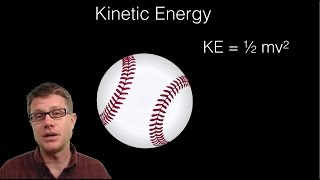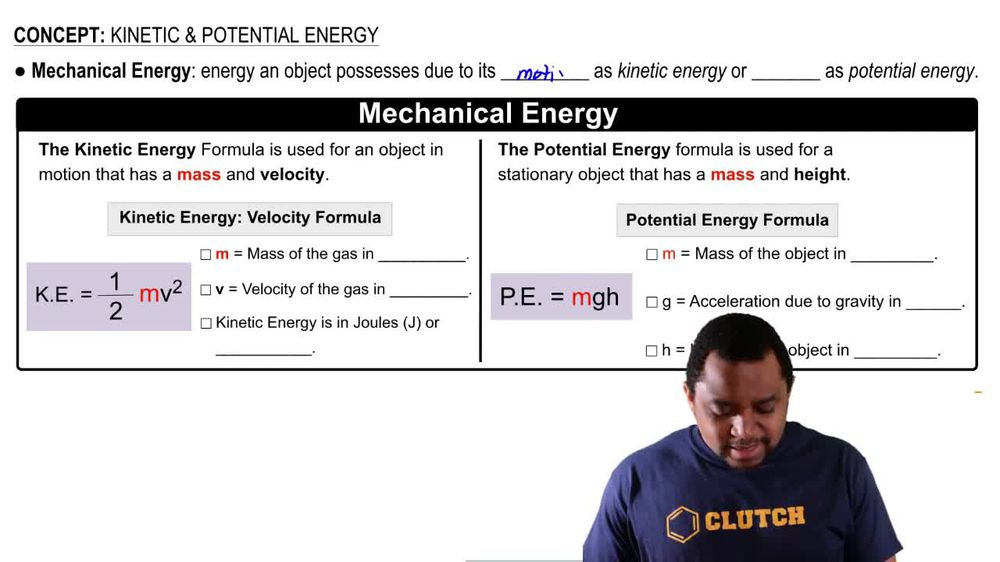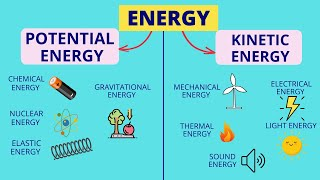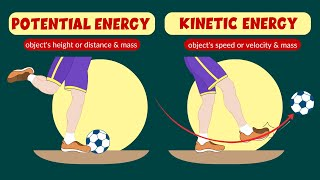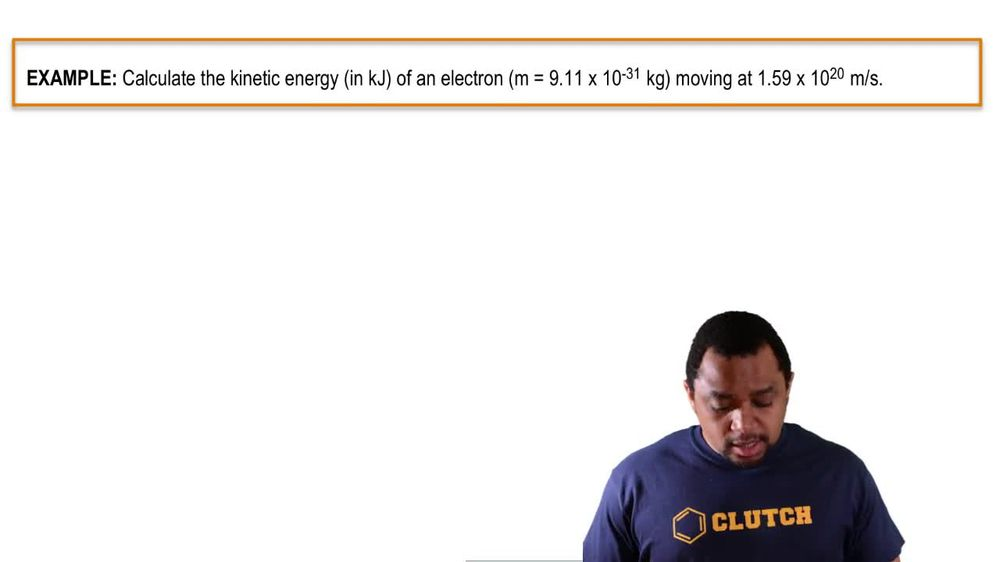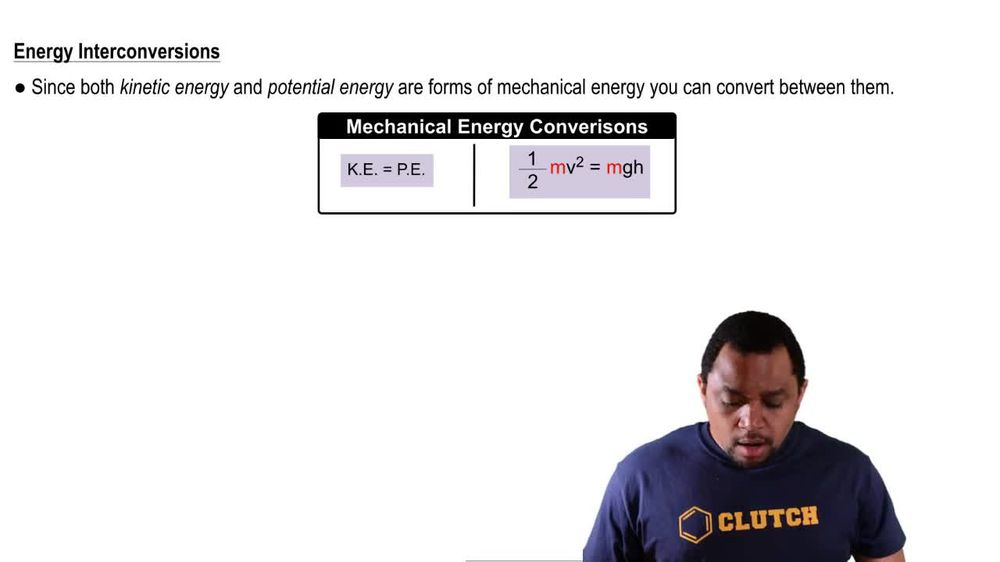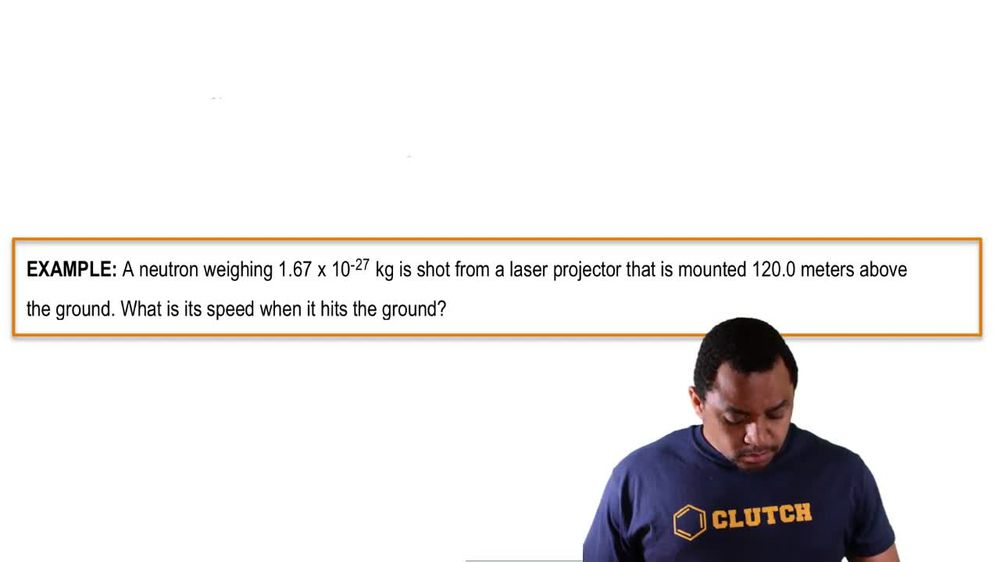Start typing, then use the up and down arrows to select an option from the list.
1. 8. Thermochemistry2. Kinetic & Potential Energy# Kinetic & Potential Energy Example 1

by Jules Bruno
200 views
3
0
here we need to calculate the kinetic energy in kill jewels of an electron which has a mass of 9.11 times 10 to the negative, 31 kg moving at 1.59 times 10 to the m per second. Remember, kinetic energy equals half times maths times velocity squared here are mass is in kilograms. So we're good times a velocity which is in meters per second. So that's also good. And then we know that we have to square this now when I square that what does it really come out? Thio? So I'm squaring everything inside of here that comes out to 2.5 to times 10 of the 40 m squared over second squared. So we have kilograms times meter squared over second squared. So that's 1.15 times 10 to the 10 kg times meter squared over second squared which remember, is the same thing as jewels. So 1.15 times 10 to the 10 jewels will be the kinetic energy of this particular electron. Here we have three sig figs in our answer because the values given to us when within the question also have three significant figures